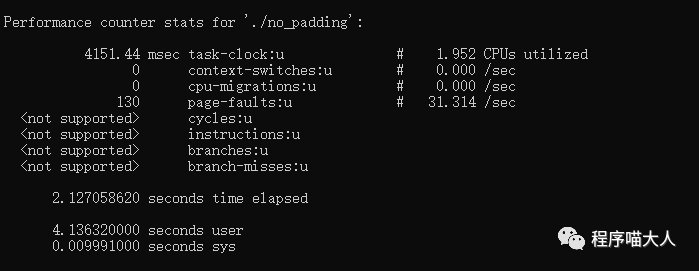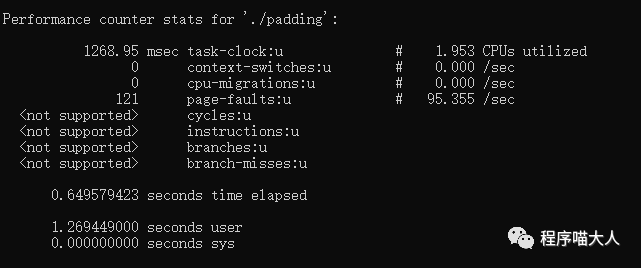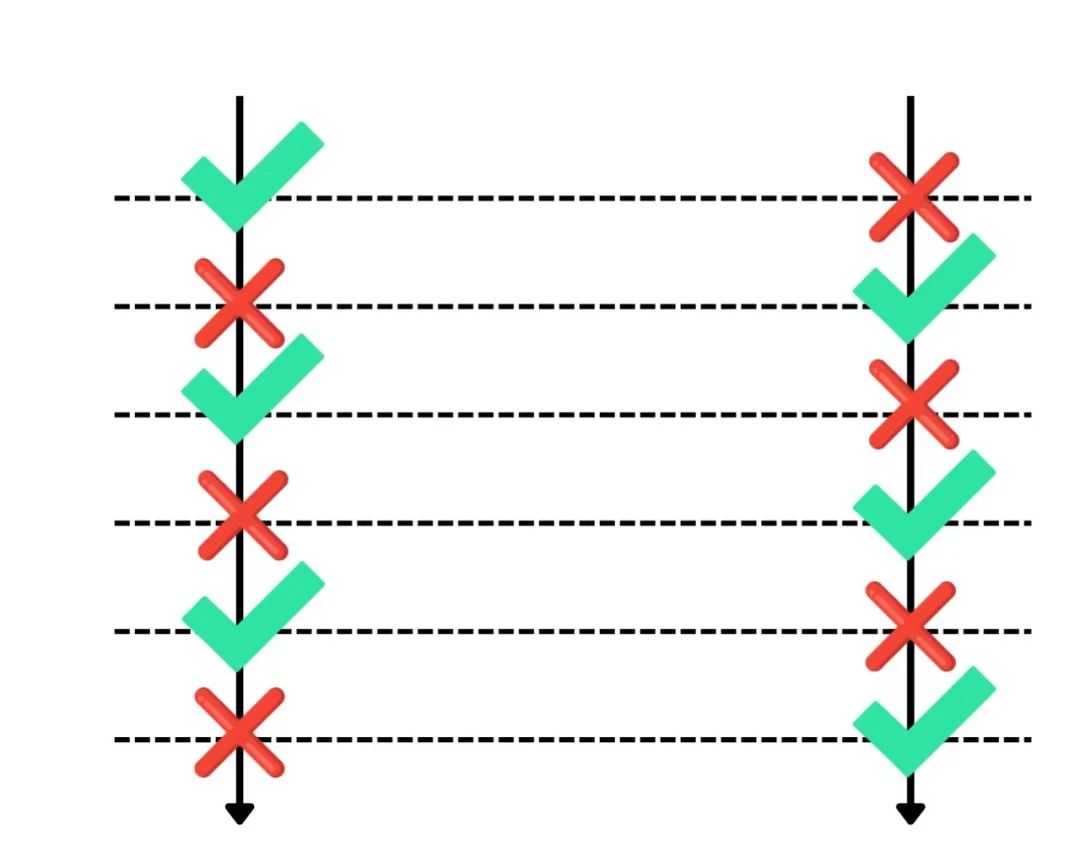# CPU Cache伪共享问题

85次阅读  |  发布于5月以前

``````
const int row = 10240;
const int col = 10240;
int matrix[row][col];
int TestRow() {
//按行遍历
int sum_row = 0;
for (int r = 0; r < row; r++) {
for (int c = 0; c < col; c++) {
sum_row += matrix[r][c];
}
}
return sum_row;
}``````

``````
int TestCol() {
//按列遍历
int sum_col = 0;
for (int c = 0; c < col; c++) {
for (int r = 0; r < row; r++) {
sum_col += matrix[r][c];
}
}
return sum_col;
}``````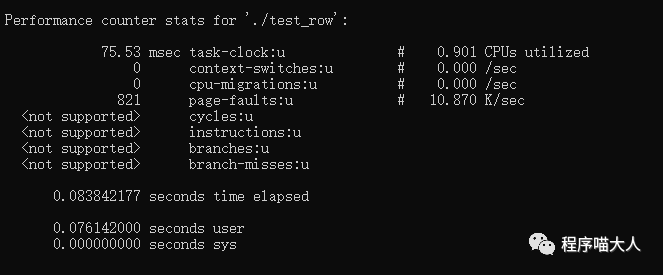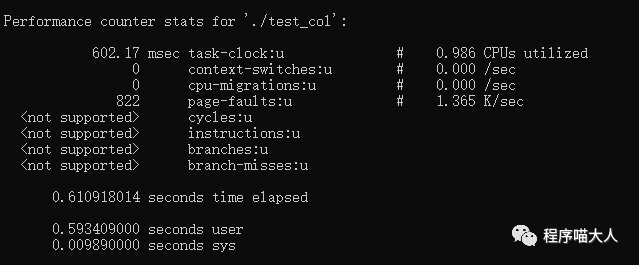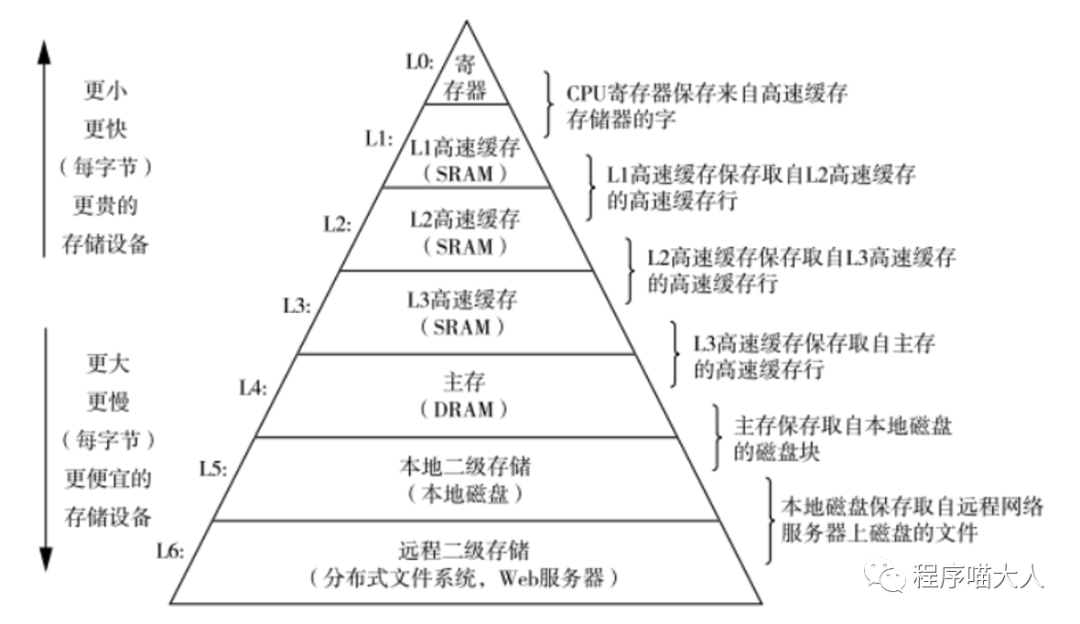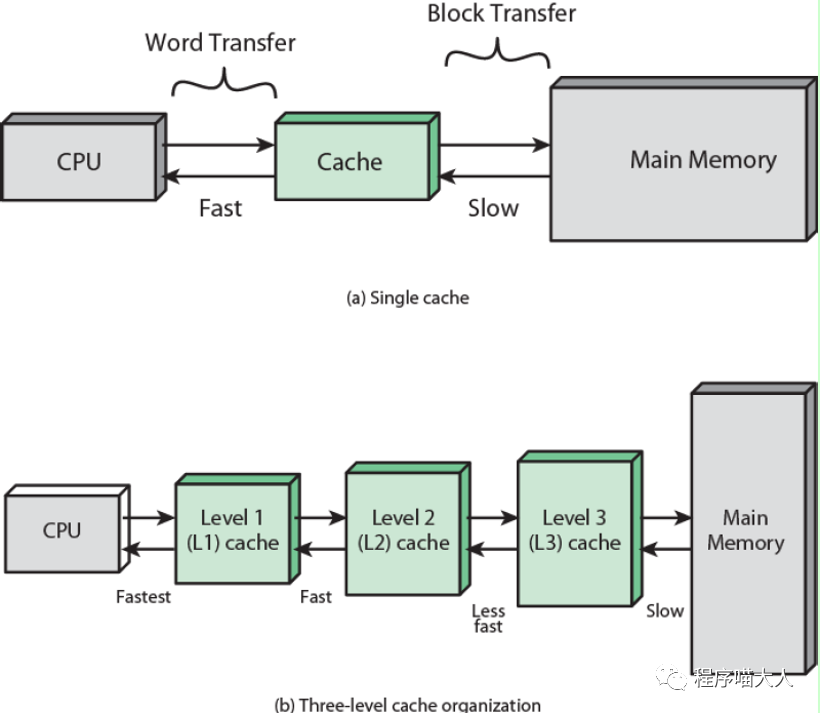``````
struct Point {
std::atomic<int> x;
// char a;
std::atomic<int> y;
};
void Test() {
Point point;
[](Point *point) {
for (int i = 0; i < 100000000; ++i) {
point->x += 1;
}
},
&point);
[](Point *point) {
for (int i = 0; i < 100000000; ++i) {
point->y += 1;
}
},
&point);
t1.join();
t2.join();
}``````

``````
struct Point {
std::atomic<int> x;
char a;
std::atomic<int> y;
};
void Test() {
Point point;
[](Point *point) {
for (int i = 0; i < 100000000; ++i) {
point->x += 1;
}
},
&point);
[](Point *point) {
for (int i = 0; i < 100000000; ++i) {
point->y += 1;
}
},
&point);
t1.join();
t2.join();
}``````